# Mitscherlich's Law: Generalization with several Fertilizers

## Abstract

It is shown, that the crop-yield z in dependence on two fertilizers x and y is the product of two components: z in dependence on x alone and z in dependence on y alone, divided by c, the yield without extern fertilizers, i.e. with x = y = 0. For n fertilizers, we have the product of n components, divided by cn-1.

## Introduction

If only one fertilizer x is used, the dependence of yield z(x) on x first was given by Mitscherlich (1909) in form of the differential equation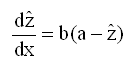(1)

where a is the asymptotic value of z, b the factor of proportionality. As usual in statistics,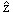is the hypothetical value, z the experimental value of the crop-yield. For equation (1) it is assumed: No over-fertilization. For the case of overfertilization see Schneeberger (2009b). Solution of formula (1) with boundary condition(x=0) = c is Mitscherlich's curve in the especial instructive form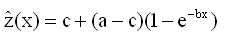(2a)

demonstrated in figure 1 with c=0.5, a=1.2, b=0,75. For estimation of the parameters in practice see Schneeberger (2009a).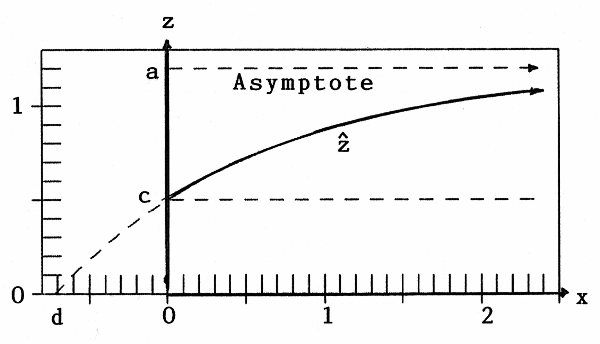Figure 1:Crop-yieldas function of one fertilizer x

An other form of formula (2a) is: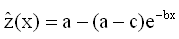(2b)

used in the following.

Baule (1918) gave the solution of equation (1) in the form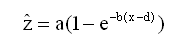(3a)

with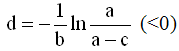(3b)

in figure 1 we have d=-0.719.

## Generalization

Now we assume two variables (=fertilizers) x and y (see figure 2)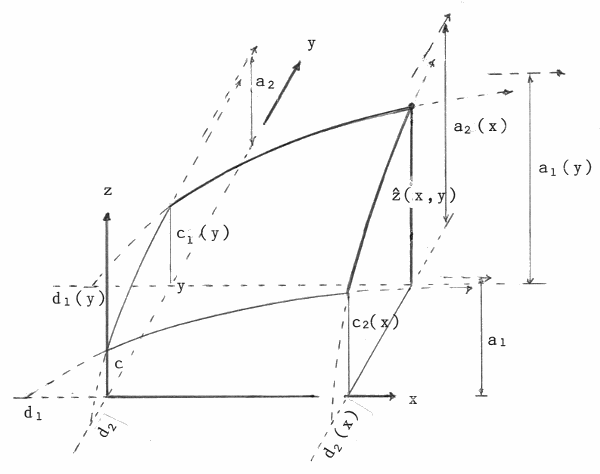Figure 2:Crop-yield(x,y) as function of two fertilizers x and y

Then we have for the crop-yield(x,y) with formula (2b)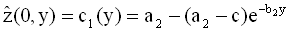(4a)

and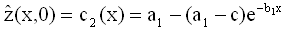(4b)

Note: For short we write

a1(y = 0) = a1, a2(x = 0) = a2, c1(y = 0) = c2 (x = 0) = c, b1(y = 0) = b1, b2(x = 0) = b2, d1(y = 0) = d1, d2(x = 0) = d2

Generalizing formulae (4) we have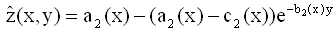(5a)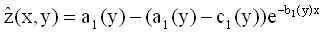(5b)

and herewith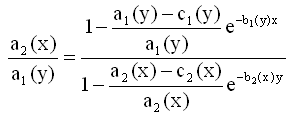(6)

Now I make use of a result of Mitscherlich (1947): ..."given different fertilizers, the parameter c (in Mitscherlich's notation) is constant for a fixed fertilizer, as I could prove in tens of years of work"... Mitscherlich's "Wirkungsgrad" c is our parameter b except for the constant ln10. This means:

b1(y) = b1 and b2(x)=b2, indepentent of y resp. x  (7)

Herewith we get from formula (6):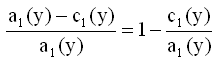is indepentent of y, i.e.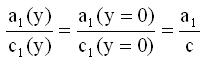(8a)

and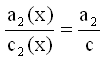independent of x (8b)

and with this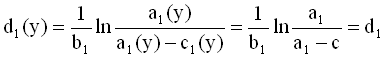independent of y (9a)

and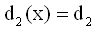independent of x (9b)

Finally we have from formula (5a) (or (5b)):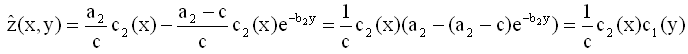(10)

or in the most instructive form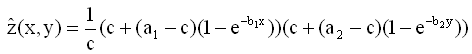(11)

Result: The generalized Mitscherlich formula in two variables is the product of the one-dimensional formulae, multiplied by 1/c.

With formulae (9) one can show, that formula (11) is identical with the formula of Baule (1918).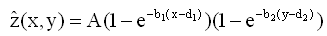with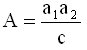(12)

Equations (10) and (11) can easily be generalized for n fertilizers: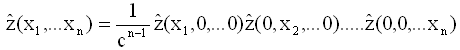(13)

## Application

The following data are from an example of Steinhauser, Langbehn and Peters (1992) with x (in 100 kg/ha of P2O5 ), y (in 100 kg/ha of K2O, z (in 1000 kg/ha of rye).

Table 1: Crop-yield(x,y) in 1000 kg/ha of rye, x in 100 kg/ha of P2O5, y in 100 kg/ha of K2O
x1 = 0.25 x2 = 0.50 x3 = 0.75 x4 = 1.00 x5 = 1.25 x6 = 1.50
y1 = 0.25 1.00 1.22 1.41 1.58 1.73 1.87
y2 = 0.50 1.41 1.79 2.09 2.34 2.55 2.73
y3 = 0.75 1.71 2.21 2.59 2.90 3.15 3.35
y4 = 1.00 2.00 2.55 2.98 3.32 3.59 3.81
y5 = 1.25 2.22 2.82 3.29 3.65 3.94 4.18
y6 = 1.50 2.41 3.05 3.55 3.93 4.24 4.50

The 5 parameters were determined with the method of Least Squares of Gauss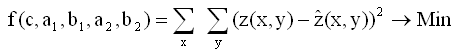(14)

summing over all 36 data-points. The minimum was gained iteratively with the non-linear Simplex-Method of Nelder and Mead (1965). The result is

c = 0.2715, a1 = 0.9472, b1 = 0.899, a2 = 1.9438, b2 = 1.027  and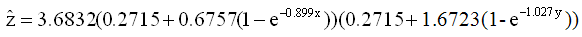In figure 3a the contour-lines(x,y)=0.2, 0.4, ... 4.0 are drawn, in figure 3b intersecting curves(x,y = const.) for y=d2, 0, 0.5, 1.0, 1.5 and ∞ are plotted. It is obvious that they are Mitscherlich-curves. We have d1= -0.376, d2 = -0.146. The asymptotes of the curves(x,y = c) are horizontal dotted straight lines in figure 3b. Especially for y = ∞ we get(∞, ∞)=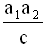= 6.78, Baule's parameter A in formula (12).(x,y = ∞) is curve a2(x) of figure 2.

In analogy intersecting curves for x=const. could be plotted.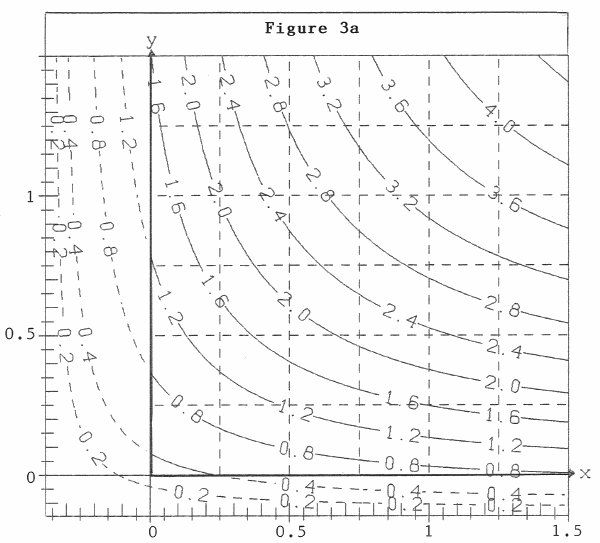Figure 3a: Contour-lines(x,y)=const. - in 1000 kg/ha of rye, x in 100 kg/ha of P2O5, y in 100 kg/ha of K2O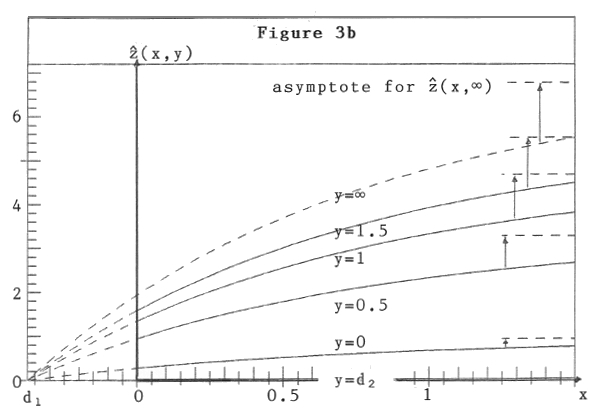Figure 3b: Mitscherlich-curves(x,y =const).

Example: With fertilizer 100 kg/ha of P2O5 (x = 1) and 50 kg/ha of K2O (y = 0.5) we get the crop-yield 2.335 kg/ha of rye (= 2.335). With x = y = 0 ( i.e. without external fertilizers) we would get 272 kg/ha of rye (= c = 0.272)

## Acknowledgement

I have to thank Dr. Embacher, Munich, who helped me to publish these papers in the internet.

A generalization with overfertilization is given in paper 5 (Paper 5: Mitscherlich's Law: Generalization with several Fertilizers and Overfertilization)

## References

Baule B. (1918). Zu Mitscherlichs Gesetz der physiologischen Beziehungen, Landwirtschaftliche Jahrbücher 51, 363-385

Mitscherlich E.A. (1909). Das Gesetz des Minimums und das Gesetz des abnehmenden Bodenertrags, Landwirtschaftliche Jahrbücher 38, 537-552

Mitscherlich E.A. (1947). Das Ergebnis von über 27000 Feld-Düngungsversuchen, Zeitschrift für Pflanzenernährung, Düngung, Bodenkunde 38. Band, 1947, Verlag Chemie

Nelder J.R. and Mead R. (1965). A Simplex Method for function minimization. The Computer Journal 7, 303-313

Schneeberger H. (2009a). Mitscherlich's Law: Sum of two Exponential Processes. Conclusions. Internet: www.soil-statistic.de, paper 1 and paper 2

Schneeberger H. (2009b). Over-Fertilization: An Inverse Mitscherlich Process. Internet: www.soil-statistic.de, paper 3

Steinhauser H., Langbehn C. and Peters U. (1992). Einführung in die landwirtschaftliche Betriebslehre, Band 1, 5.Auflage

Download this Paper in PDF format: Mitscherlich's Law: Generalization with several Fertilizers